# SSAT Upper Level Math : How to find a fraction from a percentage

## Example Questions

2 Next →

### Example Question #421 : Rational Numbers

What is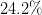as a fraction?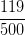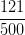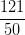Explanation:

Put the number over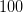and drop the percent sign.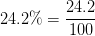Multiply the numerator and denominator by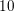to get rid of the decimal.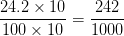Finally, reduce the fraction to get it in simplest form.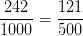### Example Question #422 : Rational Numbers

What is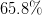as a fraction?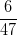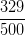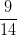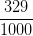Explanation:

Put the number overand drop the percent sign.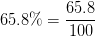Multiply the numerator and denominator byto get rid of the decimal.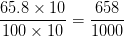Finally, reduce the fraction to get it in simplest form.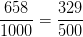### Example Question #423 : Rational Numbers

What is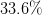as a fraction?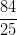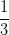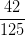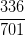Explanation:

Put the number overand drop the percent sign.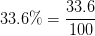Multiply the numerator and denominator byto get rid of the decimal.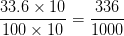Finally, reduce the fraction to get it in simplest form.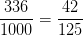2 Next →

### All SSAT Upper Level Math Resources